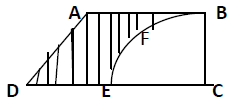mycollegehive
From a thin metallic piece, in the shape of a trapezium ABCD in which AB II CD and ∟BCD = 90˚, a quarter circle BFEC is removed. Given AB = BC = 3.5 cm and DE = 2cm, calculate the area of the remaining (shaded) part of the metal sheet (π = 22/7)
CBSE class-10 math areas-related-to-circles0From a thin metallic piece, in the shape of a trapezium ABCD in which AB II CD and ∟BCD = 90˚, a quarter circle BFEC is removed. Given AB = BC = 3.5 cm and DE = 2cm, calculate the area of the remaining (shaded) part of the metal sheet (π = 22/7)20 viewsShareFollowUniversity of Benin Nigeria
01 August 2020University of Lagos Nigeria
01 August 20200Shape ABCD is a parallelogram, and the area of a parallelogram = 1/2 x (sum of parallel sides) x height

parallel sides = AB and CD

height = BC

Thus, area of the parallelogram = 1/2 x (AB + CD) x BC

BFEC is a quarter circle, and the area of a quarter circle = πr2/4

Also, the radius of the quarter = BC. That is,

r = BC

Thus, area of the quarter circle = π(BC)2/4

since the quarter is removed, thus, area of the remaining (shaded) part = Area of trapenzium - Area of quarter circle

= [1/2 x (AB + CD) x BC] - [π(BC)2/4]

AB = 3.5cm

BC = 3.5cm

CD = DE + EC

EC = BC

Thus, CD = DE + BC = 2 + 3.5 = 5.5cm

Therefore, area of the remaining (shaded) part = [1/2 x (3.5 + 5.5) x 3.5] - [π(3.5)2/4]

= [1/2 x 9 x 3.5] - [49π/16]

but π = 22/7

= [63/4] - [49/16 x 22/7]

= = [63/4] - [77/8]

= 49/8

= 6.125 cm2Share### Related Tags

CBSE

0 followers

56 questionsclass-10

0 followers

56 questionsmath

2 followers

985 questions